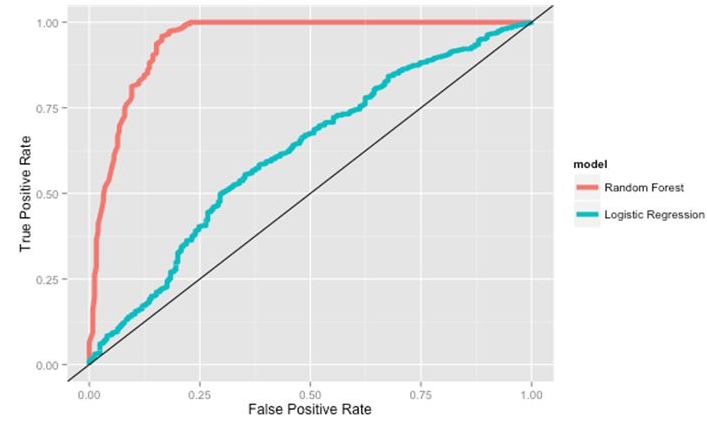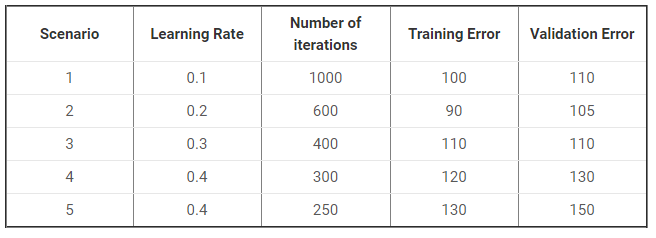Machine Learning Masterclass scholarship test:
Referral code
Whatsapp Num *
College Name *
Which of the following is a supervised learning algorithm ?
Which of the following machine learning algorithm is best suited for prediction of house prices ?
Which of the following is/are example of ensemble learning ?
Random forest is an example of ______ ?
Suppose you have 100 decision trees in your random forest model with x1, x2, x3 .... x100 accuracies respectively. What will be the accuracy of the random forest for classification task ?
Suppose there are 10 relevant movies for a user.If you make a recommendation system which recommends 7 movies to that user in which only 3 are relevant then what is the recall ?
Suppose there are 10 relevant movies for a user.If you make a recommendation system which recommends 7 movies to that user in which only 3 are relevant then what is the precision ?
Suppose you have 100 decision trees in your random forest model with x1, x2, x3 .... x100 accuracies respectively. What will be the accuracy of the random forest for regression task ?
Suppose you are working on a multiple regression problem where you have 10 features.If you want to regularize your linear regression model knowing the fact that all the features are important which of the following regularization would you use ?
In ______, individual trees are independent of each other._______ is the method for improving the performance by aggregating the results of weak learners ??
Which of the following algorithm would you choose for final model building based on the below performance graph ?Which of the following algorithm is based on bootstrap aggregation ?
In bagging each individual tree has ___ variance and ____ bias ?
What if we set C parameter to infinite in SVM ?
Which of the following algorithm has smallest training time ?
Which of the following parameter in SVM is responsible for tradeoff between misclassification and simplicity of model ?
Suppose you have been given the following scenario for training and validation error for Linear Regression.Which of the following scenario would give you the right hyper parameter ?